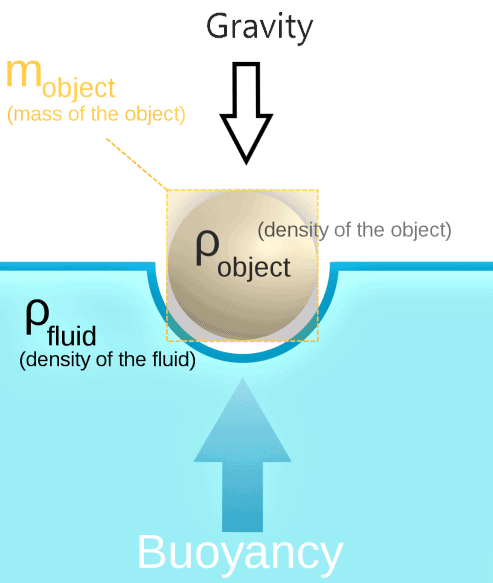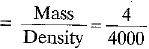Courses

# NCERT Exemplar - Gravitation (Floatation) ( PART - B) Class 9 Notes | EduRev

## Class 9 : NCERT Exemplar - Gravitation (Floatation) ( PART - B) Class 9 Notes | EduRev

The document NCERT Exemplar - Gravitation (Floatation) ( PART - B) Class 9 Notes | EduRev is a part of Class 9 category.
All you need of Class 9 at this link: Class 9

Q.1. An object weighs 10 N in air. When immersed fully in water, it weighs 8 N. The weight of the liquid displaced by the object will be:
(a) 2 N
(b) 8 N
(c) 10N
(d) 12 N
Ans: Weight in water = 8 N,
Weight in air - 10 N
Weight of liquid displaced = Loss in weight
= 10 N - 8 N = 2 N

Q.2. An object is put one by one in three liquids having different densities. The object floats with 1/9, 2/11 and 3/7 parts of their volumes outside the liquid surface in liquids of densities d1, d2 and d3 respectively. Which of the following statement is correct?
(a) d1 > d2 > d3
(b) d1 > d2 < d3
(c) d1 < d2 > d3
(d) d1 < d2 < d3
Ans: Volume of solids above water surface = 1/9, 2/11 and 3/7 respectively.
Volume of solids immersed = 1 - (1/9),
1 - (2/11) and 1 - (3/7) respectively,
i.e. 8/9, 9/11 and 4/7 respectively.As V1 > V2 > V3, the densities of liquids are in magnitude d1 < d2 < d3 This is because, lesser the volume of solid immersed, more is the upthrust acting on it and hence, greater is the density of fluid.

Q.3. (a) A cube of side 5 cm is immersed in water and then in saturated salt solution. In which case will it experience a greater buoyant force? If each side of the cube is reduced to 4 cm and then immersed in water, what will be the effect on the buoyant force experienced by the cube as compared to the first case? Give reason for each case.
(b) A ball weighing 4 kg of density 4000 kgm-3 is completely immersed in water of density 103 kgm-3. Find the force of buoyancy on it. (Given g = 10 ms-2)
Ans: (a) The cube experiences a greater buoyant force when immersed in salty water because salty water has greater density than water. When the side of cube is reduced, then the buoyant force acting on it due to water, will reduce. This is according to Archimedes’ principle as when the volume of a body is less, an upthrust exerted by the fluid on it is also less.
(b) Given: Mass of ball = 4 kg,
Density of ball = 4000 kg/m3,
Diversity of water = 103 kgm-3,g = 10 ms-2
Volume of ball, V= 10-3 m3
Upthrust, U = Vρg = 10-3 x 10x 10
= 10 N

Offer running on EduRev: Apply code STAYHOME200 to get INR 200 off on our premium plan EduRev Infinity!

,

,

,

,

,

,

,

,

,

,

,

,

,

,

,

,

,

,

,

,

,

;